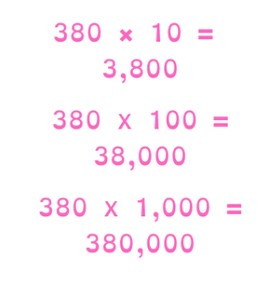Multiplying by 10, 100 or 1,000

# Multiplying by 10, 100 or 1,000

Students learn to multiply by 10, 100, and 1,000.8,000 schools use Gynzy92,000 teachers use Gynzy1,600,000 students use Gynzy

## General

The students learn to multiply by 10, 100, and 1,000.

## Standards

CCSS.Math.Content.4.NBT.A.1

## Learning objective

Students will be able to multiply by the numbers 10, 100, and 1,000.

## Introduction

Have the students listen to the number and write the numbers in the correct places in the HTO chart.

## Instruction

Explain that you can multiply by 10 by putting a zero at the end of the answer. With multiplication by 100, two zeros are added at the end of the answer. If you are multiplying by 1,000, then three zeros are added at the end of the answer. Have the students discuss in pairs how many zeros they would add at the end of the answer. Next you show a few problems in which paint is covering different parts of the problem. Ask the students what numbers are covered by the paint and drag the paint spots away to check whether they are correct. Afterwards, you have the students solve a few problems and ask how they did it. Finally, you show how you can solve a story problem. Have the students solve the next two story problems on their own.

Check whether the students can multiply by 10, 100, and 1,000 with the following question:
- How do you solve 1000 × 375?

## Quiz

The students test their understanding of multiplying by 10, 100, and 1,000 through ten exercises. Some of these are abstract problems, and others are story problems.

## Closing

You discuss with the students once again that it is important to be able to multiply by 10, 100, and 1,000, because that is how you can solve multiplication problems with large numbers. As a closing activity you show a parking lot. In the parking lot there are multiplication problems, and on each car there is an answer. Drag the car to the correct problem. Afterwards, you spin the three wheels. You fill the numbers in on the lines. Then there is a problem that the students must solve.

## Teaching tips

Have students that have difficulty with multiplying by 10, 100 and 1,000 first practice multiplying by 10. Then they can practice multiplying by 100. Emphasize that there are now two zeros that are added to the end of the answer. Finally they can practice multiplying with 1,000, for which three zeros are added at the end of the answer.

### The online teaching platform for interactive whiteboards and displays in schools

• Save time building lessons

• Manage the classroom more efficiently

• Increase student engagement